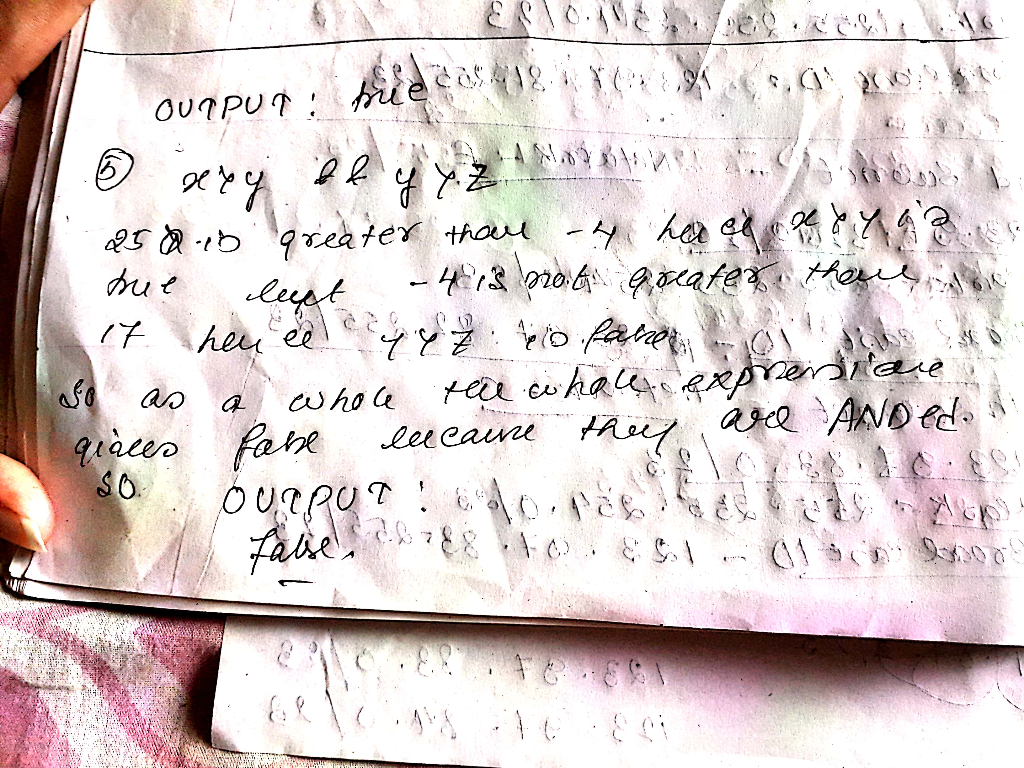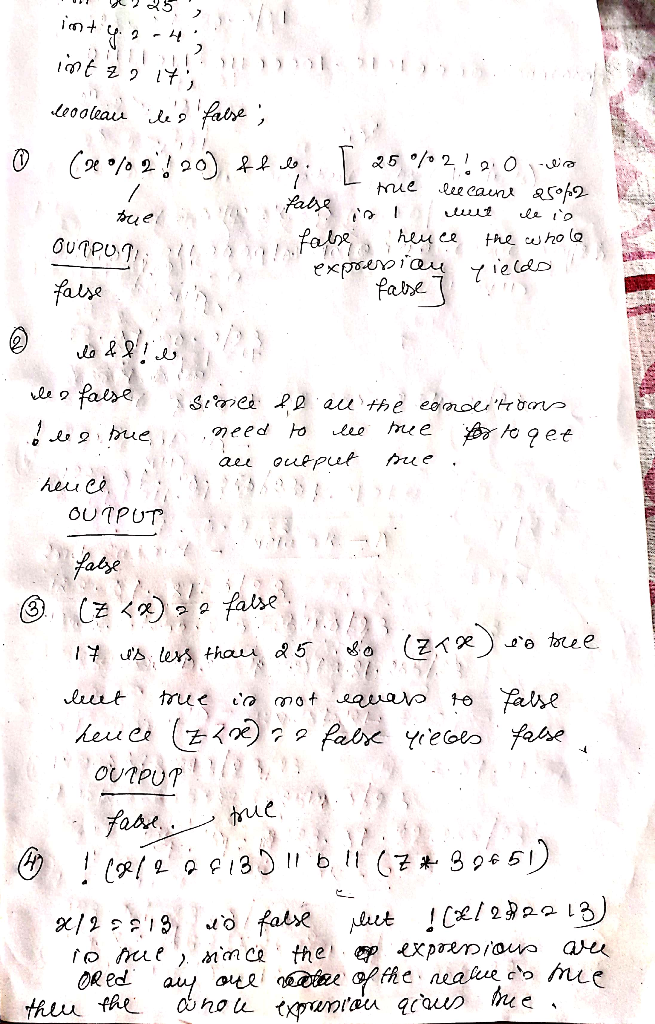# Provide the expected output AS IT WOULD APPEAR IN A JAVA PROGRAM as the result of a print statement. (i.e. Enter true or...

Provide the expected output AS IT WOULD APPEAR IN A JAVA PROGRAM as the result of a print statement.

(i.e. Enter true or false (all lower case letters). If you enter TRUE, F, True, etc these will be marked wrong).

Given the following declarations:

int x = 25;

int y = -4;

int z = 17;

boolean b = false;

What would be the output of the following Boolean expressions?

(x % 2 != 0) && b

b && !b

(z < x) == false

!(x / 2 == 13) || b || (z * 3 == 51)

x > y && y > z##### Add Answer of: Provide the expected output AS IT WOULD APPEAR IN A JAVA PROGRAM as the result of a print statement. (i.e. Enter true or...
More Homework Help Questions Additional questions in this topic.